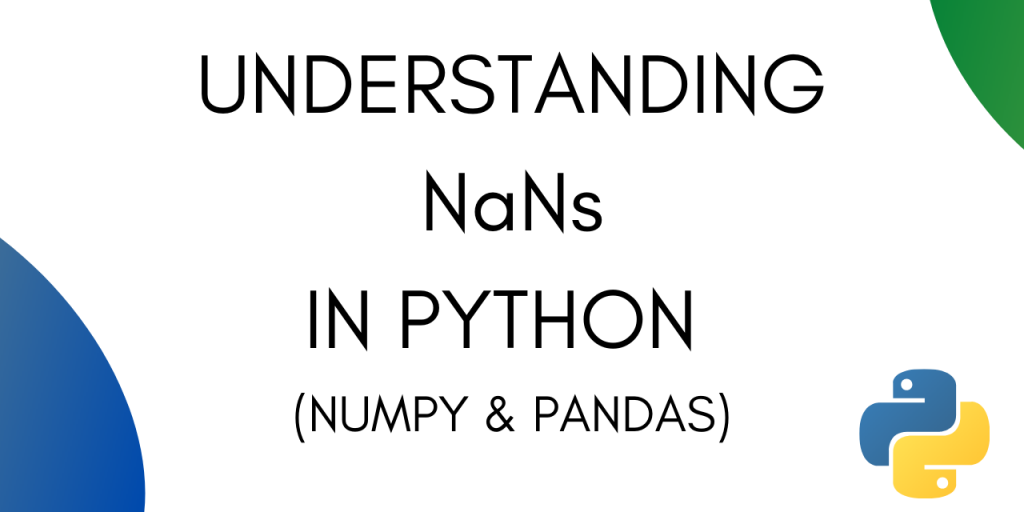# Understanding NaN in Numpy and PandasNaN is short for Not a number. It is used to represent entries that are undefined. It is also used for representing missing values in a dataset.

The concept of NaN existed even before Python was created. IEEE Standard for Floating-Point Arithmetic (IEEE 754) introduced NaN in 1985.

NaN is a special floating-point value which cannot be converted to any other type than float.

In this tutorial we will look at how NaN works in Pandas and Numpy.

## NaN in Numpy

Let’s see how NaN works under Numpy. To observe the properties of NaN let’s create a Numpy array with NaN values.

```import numpy as np
arr = np.array([1, np.nan, 3, 4, 5, 6, np.nan])
pritn(arr)
```

Output :

```[ 1. nan  3.  4.  5.  6. nan]
```

### 1. Mathematical operations on a Numpy array with NaN

Let’s try calling some basic functions on the Numpy array.

```print(arr.sum())
```

Output :

```nan
```

Let’ try finding the maximum from the array :

```print(arr.max())
```

Output :

```nan
```

Thankfully Numpy offers methods that ignore the NaN values while performing Mathematical operations.

### 2. How to ignore NaN values while performing Mathematical operations on a Numpy array

Numpy offers you methods like np.nansum() and np.nanmax() to calculate sum and max after ignoring NaN values in the array.

```np.nansum(arr)
```

Output :

```19.0
```
```np.nanmax(arr)
```
```6.0
```

If you have your autocompletion on in your IDE, you will see the following list of options while working with np.nan :

### 3. Checking for NaN values

To check for NaN values in a Numpy array you can use the np.isnan() method.

This outputs a boolean mask of the size that of the original array.

```np.isnan(arr)
```

Output :

```[False  True False False False False  True]
```

The output array has true for the indices which are NaNs in the original array and false for the rest.

### 4. Equating two nans

Are two NaNs equal to one another?

This can be a confusing question. Let’s try to answer it by running some python code.

```a = np.nan
b = np.nan
```

These two statements initialize two variables, a and b with nan. Let’s try equating the two.

```a == b
```

Output :

```False
```

In Python we also have the is operator. Let’s try using that to compare the two variables.

```a is b
```

Output :

```True
```

The reason for this is that == operator compares the values of both the operands and checks for value equality. `is operator`, on the other hand, checks whether both the operands refer to the same object or not.

In fact, you can print out the IDs of both a and b and see that they refer to the same object.

```id(a)
```

Output :

```139836725842784
```
```id(b)
```

Output :

```139836725842784
```

## NaN in Pandas Dataframe

Pandas DataFrames are a common way of importing data into python. Let’s see how can we deal with NaN values in a Pandas Dataframe.

Let’s start by creating a dataframe.

``` s = pd.DataFrame([(0.0, np.nan, -2.0, 2.0),
...                    (np.nan, 2.0, np.nan, 1),
...                    (2.0, 5.0, np.nan, 9.0),
...                    (np.nan, 4.0, -3.0, 16.0)],
...                   columns=list('abcd'))
s
```

Output :

### 1. Checking for NaN values

You can check for NaN values by using the isnull() method. The output will be a boolean mask with dimensions that of the original dataframe.

```s.isnull()
```

Output :

### 2. Replacing NaN values

There are multiple ways to replace NaN values in a Pandas Dataframe. The most common way to do so is by using the .fillna() method.

This method requires you to specify a value to replace the NaNs with.

```s.fillna(0)
```

Output :

Alternatively, you can also mention the values column-wise. That means all the NaNs under one column will be replaced with the same value.

```values = {'a': 0, 'b': 1, 'c': 2, 'd': 3}
s.fillna(value=values)
```

Output :

You can also use interpolation to fill the missing values in a data frame. Interpolation is a slightly advanced method as compared to .fillna().

Interpolation is a technique with which you can estimate unknown data points between two known data points.

### 3. Drop rows containing NaN values

To drop the rows or columns with NaNs you can use the .dropna() method.

To drop rows with NaNs use:

```df.dropna()
```

To drop columns with NaNs use :

```df.dropna(axis='columns')
```

## Conclusion

This tutorial was about NaNs in Python. We majorly focused on dealing with NaNs in Numpy and Pandas. Hope you had fun learning with us.